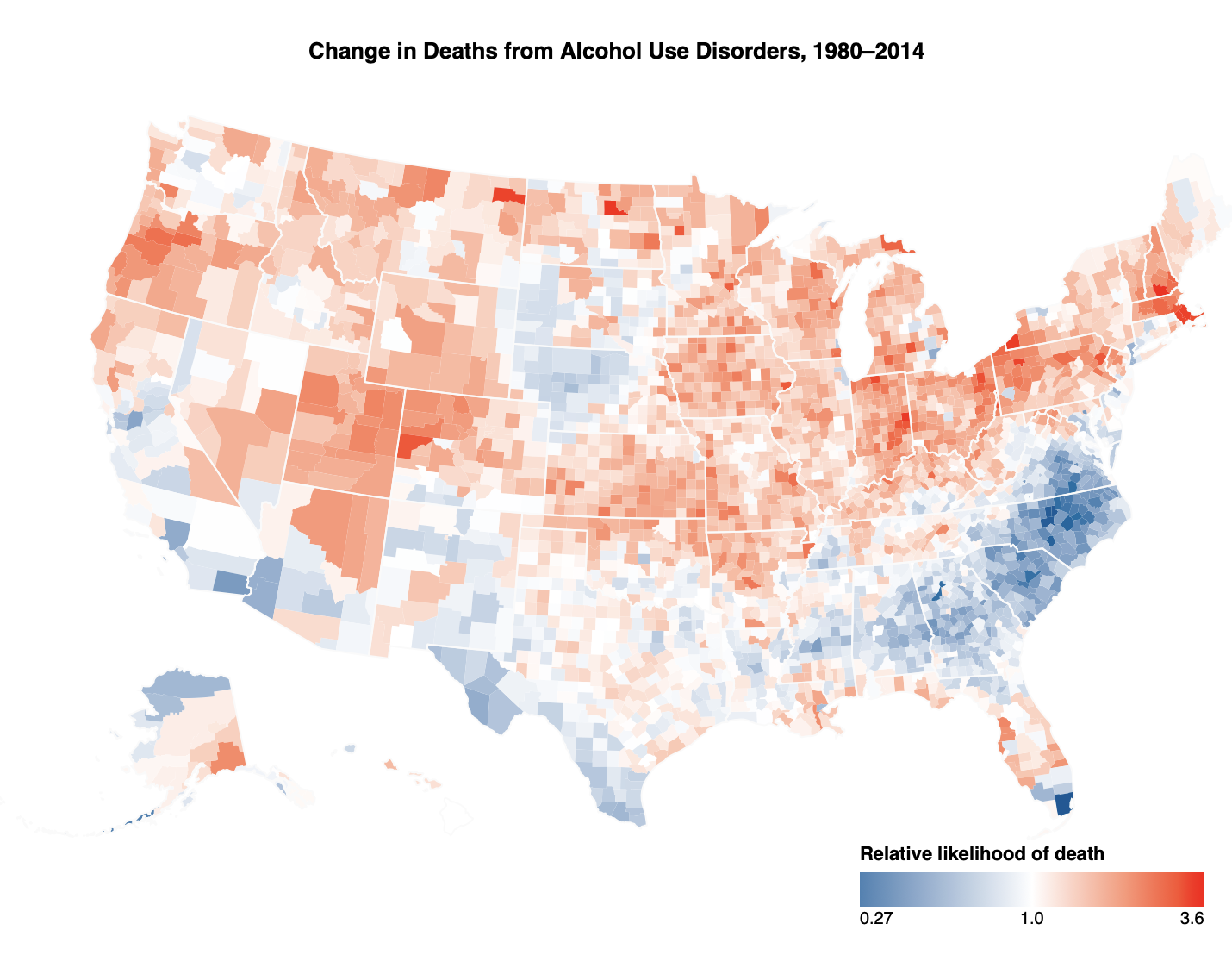Don’t compare percentage change on a linear scale; use a log scale instead. -50% (0.5×) is as big a change as +100% (2×). 比较比例变化，不要在线性尺度上，而要在对数尺度上。因为减少了 50% 与增加了 100% 是一样大的变动。

• 如何对比新旧数据之间比例的变化
• 如何展示对比结论

Lisa 行文更偏重于解释「何为 Log 刻度」以及「为什么、何时使用它」。第一篇引用了新西兰游客人数逐年变化的例子，很直观讲了对数刻度是什么以及使用它有什么好处；第二篇则引用了摩尔定律涉及的晶体管数量变化，讲了使用对数刻度的场合和需要注意的情况，比如坐标不可能从零开始等；第三篇则猜测了 Mike 原推的意思，为什么「-50%(0.5x)  也是 +100% 」以及她对百分之变化用线性还是对数坐标刻度的建议。

Mike 则综合强调了「对比和展示」。比如他以「全美滥用酒精致死情况」这一例子，2014 vs. 1980，他用了 4 种对比方式来展现经过 30 多年以后，每 10 万人中酒精滥用致死人数的变化：

• 并排做两幅图，让读者对着看
• 同一幅图展示两者差值 `b − a`
• 同一幅图展示两者相对变化 `(a - b) / a`
• 同一幅图展示两者比例变化 `a / b`，并取对数

## 动手玩

Mike Bostock 是 D3 的作者，他演示用的代码都是 D3，操作平台也是自己的新项目 Observable。我还没有玩过 D3，以下内容如有涉及绘图，采用的将会是最近在玩的 Altair（其实底层也还是 vega-lite 和 D3），跑在本地 Jupyter Notebook，或是 nteract 或是 Google Colab 上都行，没什么区别。点击 ▶︎ 展开代码。

1. 新西兰的游客
``````
import numpy as np
import pandas as pd
import altair as alt

#
# linear scale
#
alt.Chart(nzt).mark_line().encode(
x=alt.X('yearmonth(Date):T', axis=alt.Axis(format='%Y', title=None)),
y=alt.Y('Close', title=None)
).properties(
width=920
)

#
# log scale
#
alt.Chart(nzt).mark_line().encode(
x=alt.X('yearmonth(Date):T', axis=alt.Axis(format='%Y', title=None)),
y=alt.Y('Close', title=None)
).properties(
width=920
)
```
`````````In : import math

In : math.log(8/4, math.e)
Out: 0.6931471805599453

In : math.log(4/8, math.e)
Out: -0.6931471805599453
``````

``````
alt.Chart(deaths).mark_tick().encode(
color=alt.Color('ratio_change:Q', scale=alt.Scale(type='log', domain=domain, range=['steelblue', 'white', 'red']), legend=alt.Legend(orient='bottom-left', format='.2r', offset=0, title=None))
```
`````````
deaths2 = deaths[deaths['location_name'].str.contains('County')].sample(n=30)

alt.Chart(deaths2).mark_square(size=400).encode(
x=alt.X('location_name:O', title=None),
color=alt.Color('ratio_change:Q', scale=alt.Scale(type='log', domain=domain, range=['steelblue', 'white', 'red'], nice=True), legend=None)
).properties(
width=720
).configure_view(strokeWidth=0)
```
`````````
import math
import numpy as np
import pandas as pd
import altair as alt
from vega_datasets import data

counties = alt.topo_feature('./data/cb_2017_us.json', 'counties')
states = alt.topo_feature('./data/cb_2017_us.json', 'states')
deaths = pd.read_csv('./data/data-OXy0O.csv', skiprows=1, names=['fips_code', 'percent_change', 'location_name'], dtype={'fips_code': 'str', 'percent_change': np.float64, 'location_name': 'str'})

deaths['ratio_change'] = deaths['percent_change'].apply(lambda x: (x + 100) / 100)

max_ratio = min(max(temp['2014'] / temp['1980']), max(temp['1980'] / temp['2014']))
domain = [(1 / max_ratio), 1, max_ratio]
title = 'Change in Deaths from Alcohol Use Disorders, 1980–2014'

counties_choropleth = alt.Chart(counties).mark_geoshape().encode(
color=alt.Color(
'ratio_change:Q',
scale=alt.Scale(type='log', domain=domain, range=['steelblue', 'white', 'red'], interpolate='lab', zero=False),
legend=alt.Legend(orient='bottom-right', title='Relative likelihood of death', format='.2r')
),
tooltip=alt.Tooltip('location_name:O')
).transform_lookup(
lookup='properties.GEOID',
from_=alt.LookupData(deaths, 'fips_code', ['ratio_change', 'location_name'])
).project(
type='albersUsa'
).properties(
width=720,
height=560,
title=alt.TitleParams(title, orient='top', offset=-40)
)

states_choropleth = alt.Chart(states).mark_geoshape(filled=False, stroke='#fafafa', strokeWidth=1).encode()
death_map = counties_choropleth + states_choropleth